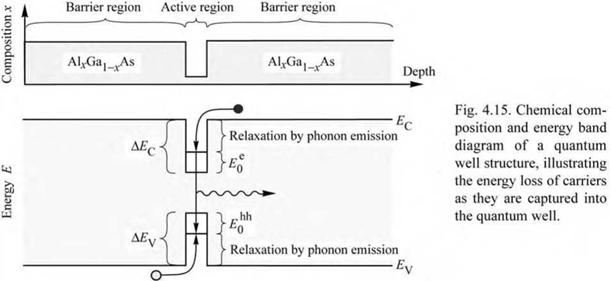## Diode voltage

The energy of an injected electron is converted into optical energy upon electron-hole recombination. Thus, conservation of energy requires that the drive voltage or forward voltage of a light-emitting device is equal to (or larger than) the bandgap energy divided by the elementary charge. The diode voltage is thus given by

V = hv/ e к Eg/ e. (4.41)

There are several mechanisms causing the drive voltage to be slightly different from this value and these mechanisms will be discussed below.

Firstly, if the diode has a significant series resistance, an additional voltage drop occurs. The additional resistance can be caused by (i) contact resistance, (ii) resistances caused by abrupt

heterostructures, and (iii) bulk resistance occurring particularly in materials with low carrier concentrations or low carrier mobilities. A voltage drop of magnitude IRs occurs at the series resistance thereby increasing the drive voltage.

Secondly, carrier energy may be lost upon injection into a quantum well structure or double heterostructure. An example of non-adiabatic injection is shown in Fig. 4.15, which shows a thin quantum well under forward-bias conditions. The figure illustrates that upon injection into the quantum well, the electron loses energy AEC - E0 , where AEC is the band discontinuity and E0 is the energy of the lowest quantized state in the conduction-band quantum well. Similarly, the energy lost by holes is given by AEV - E0 , where AEV is the band discontinuity and E0 is the energy of the lowest state in the valence-band quantum well. Upon injection of carriers into the well, the carrier energy is dissipated by phonon emission, i. e. by conversion of the carrier energy to heat. The energy loss due to non-adiabatic injection of carriers is relevant in semiconductors with large band discontinuities, for example GaN and other group-III nitride materials.

Thus, the total voltage drop across a forward-biased LED is given by

y. Eg t r> AEc — E0 AEv — E0

V = —s - + IRs + C ^ V(4.42)

e e e

where the first summand on the right-hand side of the equation is the theoretical voltage minimum, the second summand is due to the series resistance in the device, and the third and
fourth summands are due to non-adiabatic injection of carriers into the active region.One finds experimentally that the diode voltage can be slightly lower than the minimum value predicted by Eq. (4.42), i. e. can be slightly lower than Eg/ e « hv / e. Both electrons and holes carry, on average, the thermal energy kT. In a forward-biased p-n junction, high-energy carriers are more likely than low-energy carriers to diffuse over to the side of opposite conductivity type where they recombine. At room temperature, 4kT/ e amounts to a voltage of about 100 mV. In low-resistance devices, the diode voltage can be 100-200 mV lower than hv/ e. For example, in forward-biased GaAs LEDs (Eg = 1.42 eV), some photon emission with hv = 1.42 eV is observed at diode voltages of about 1.32 V, i. e. lower than the photon energy.

Exercise: Drive voltages of LEDs. Calculate the approximate forward diode voltage of LEDs emitting in the blue, green, and red parts of the visible spectrum. Also calculate the forward diode voltage of LEDs emitting at 870 nm and 1.55 ^m.

Solution:

 Wavelength 470 nm 550 nm 650 nm 870 nm 1550 nm

Emission color Blue Green Red IR IR

Photon energy

2.6 eV

2.2 eV

1.9 eV

1.4 eV 0.8 eV

Drive voltage

2.6 V

2.2 V

1.9 V

1.4 V 0.8 V

Комментарии закрыты.